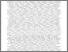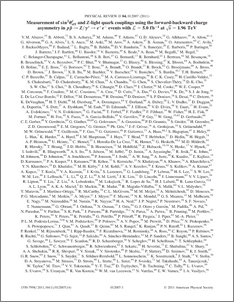# Measurement of sin^2θ_{eff}^{lept} and Z-light quark couplings using the forward-backward charge asymmetry in p\bar{p} -> Z/γ^{*} -> e^{+}e^{-} events with L=5.0 fb^{-1} at \sqrt{s}=1.96 TeV

Collaboration, D0 and Bertram, Iain and Borissov, Guennadi and Fox, Harald and Ross, Anthony and Williams, Mark and Ratoff, Peter (2011) Measurement of sin^2θ_{eff}^{lept} and Z-light quark couplings using the forward-backward charge asymmetry in p\bar{p} -> Z/γ^{*} -> e^{+}e^{-} events with L=5.0 fb^{-1} at \sqrt{s}=1.96 TeV. Physical Review D, 84 (1). ISSN 1550-7998Preview
PDF
PhysRevD.84.012007.pdf - Published Version

## Abstract

We measure the mass dependence of the forward-backward charge asymmetry in 157,553 $p\bar{p} \rightarrow Z/\gamma^{*} \rightarrow e^+e^-$ interactions, corresponding to 5.0 fb$^{-1}$ of integrated luminosity collected by the D0 experiment at the Fermilab Tevatron Collider at $\sqrt{s}=1.96$ TeV. The effective weak mixing angle ($\sin^2\theta_{eff}^{lept}$) from this process involving predominantly the first generation of quarks is extracted as $\sin^2\theta_{eff}^{lept} = 0.2309 \pm 0.0008 ({stat.}) \pm 0.0006 ({syst.})$. We also present the most precise direct measurement of the vector and axial-vector couplings of $u$ and $d$ quarks to the $Z$ boson.

Item Type:
Journal Article
Journal or Publication Title:
Physical Review D